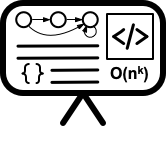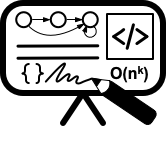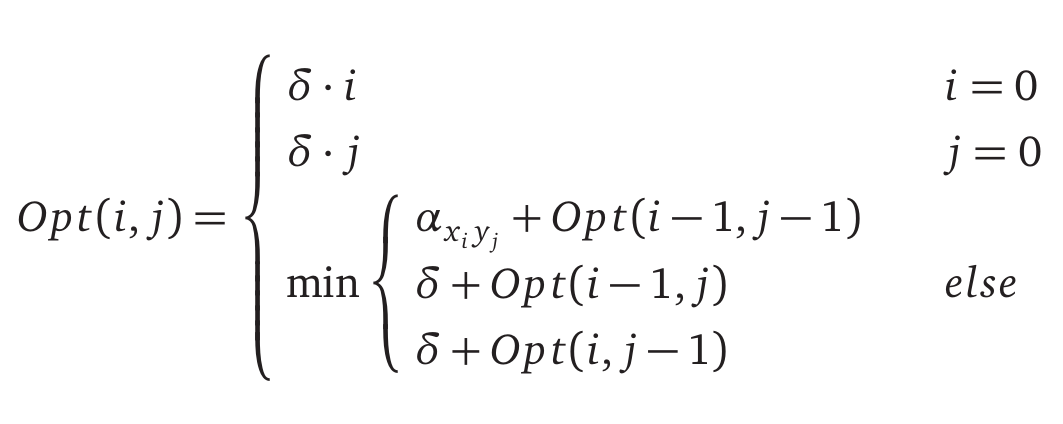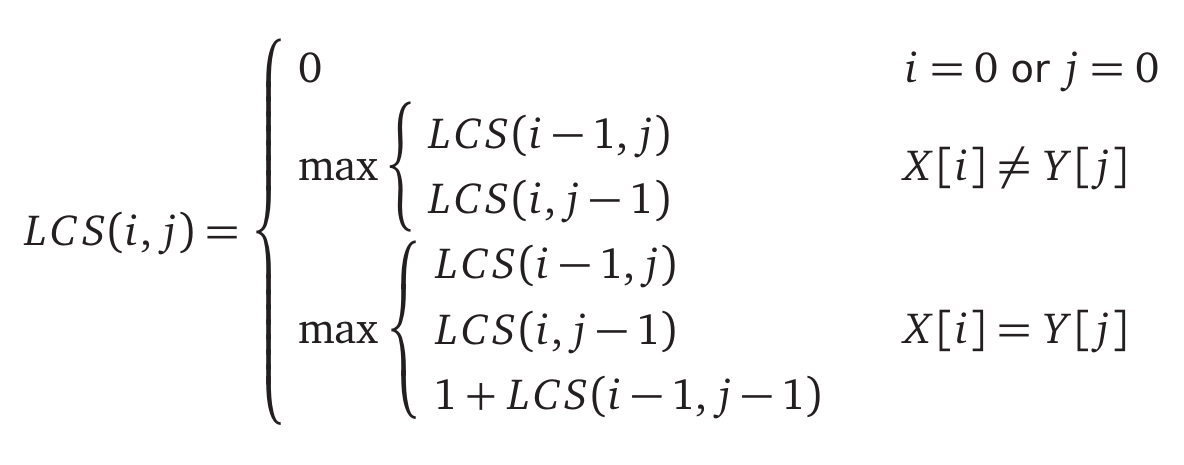# Lecture 13 - Dynamic programming II

Date Pre-lecture slides Post-lecture scribbles Lecture recording
October 10 2023## Notes

### Dynamic Programming

#### Steps:

1. Develop a recursive backtracking style algorithm, $A$, for the given problem

2. Identify the structure of the subproblems generated by $A$ on an instance, $I$, of size $n$
• Estimate the number of different subproblems as a function of $n$ (i.e. polynomial, exponential, etc)
• If the number of subproblems is small (polynomial) then there is typically a “clean” structure
3. Rewrite the subproblems in a compact fashion

4. Rewrite the recursive algorithm in terms of notation for subproblems

5. Convert $A$ to an iterative algorithm by bottom up evaluation in an appropriate order

6. Optimize further with data structures and/or additional ideas

#### Problem 1: Minimum Alignment

Background: An Alignment between two strings $X$ and $Y$ is placing one word on top of another word with potential gaps in between letters. Gaps in the first word indicate letter insertions, gaps in the second word indicate letter deletions.Problem Statement: For each mismatch in our alignment, for some $p$ and $q$ in the alphabet, we have a Mismatch Cost $\alpha_{pq}$. For each gap in our alignment we have a Gap Cost $\delta$. Given two words $X$ and $Y$ of sizes $m$ and $n$ respectively, find the alignment with the smallest cost.

1. The recursive backtracking algorithm is $Opt(i,j)$, the smallest alignment cost between strings $x_1 … x_i$ and $y_1 … y_j$. We can either insert, delete, or mismatch the last letter in the strings, the minimum alignment is the minimum of these options plus the minimum of the remaining alignment. This yields the following recurrence1. Each subproblem reduces the size of $X$ and/or $Y$ by 1, this means we will have at most $O(mn)$ different subproblems.

2. -5. This means the recursive backtracking algorithm can be implemented by filling out an array size $m+1$x$n+1$ by initializing the base cases and computing new array elements by the minimum between previously computed elements.

EDIST(A[1..m],B[1..n])
int M[0..m][0..n]
for i ← 1 to m
M[i] ← i*δ
for j ← 1 to n
M[j] ← j*δ
for i ← 1 to m
for j ← 1 to n
M[i][j] ← min{COST[A[i]][B[j]]+M[i-1][j-1],δ+M[i-1][j],δ+M[i][j-1]}
return M[m][n]


Running time is $O(mn)$. Space used is $O(mn)$.

1. When computing an array element, the algorithm only uses the current and previous column (or row). Therefore we can store only the current and previous column (or row). Adding this change in results in space used being $O(\min(m,n))$.

#### Problem 2: Longest Common Subsequence

Problem Statement: Find the longest common subsequence between two strings, $X$ and $Y$.

1. The recursive backtracking algorithm is $LCS(i,j)$, the longest common subsequence between strings $x_1 … x_i$ and $y_1 … y_j$. We can either choose to skip the last letter of $X$, skip the last letter of $Y$ or, if the last letters are the same, include the letters in the subsequence. This yields the following recurrence1. Each subproblem reduces the size of $X$ and/or $Y$ by 1, this means we will have at most $O(mn)$ different subproblems.

2. -5. This means the recursive backtracking algorithm can be implemented by filling out an array size $m+1$x$n+1$ by initializing the base cases and computing new array elements by the minimum between previously computed elements.

LCS(A[1..m],B[1..n])
int M[0..m][0..n]
for i ← 1 to m
M[i] ← 0
for j ← 1 to n
M[j] ← 0
for i ← 1 to m
for j ← 1 to n
K ← max{M[i-1][j],M[i][j-1]}
M[i][j] ← K
if A[i]=B[j]
M[i][j] ← max{K,1+M[i-1][j-1]}
return M[m][n]


Running time is $O(mn)$. Space used is $O(mn)$.

1. This problem can be formulated as the problem in example 1. Set the Mismatch Cost for two different letters is set to $+\infty$ and set to $1$ for two identical letters. Set the Gap Cost to $1$. The result is that the alignment will never mismatch two different letters so the longest common subsequence is the minimum alignment cost minus the number of gaps.

#### Even more DP problems!

Our very own Hamza Husain has dedicated himself to giving you even more DP problems for practice:

• Textbooks
• Erickson, Jeff. Algorithms
• Skiena, Steven. The Algorithms Design Manual
• Chapter 10.2 - Approximate String Matching
• Sedgewick, Robert and Wayne, Kevin. Algorithms (Forth Edition)
• Chapter 6 - Suffix Arrays
• Cormen, Thomas, et al. Algorithms (Forth Edition)
• Chapter 14 - Dynamic Programming
• Chapter 14.4 - Longest Common Subsequence
• Sariel’s Lecture 14